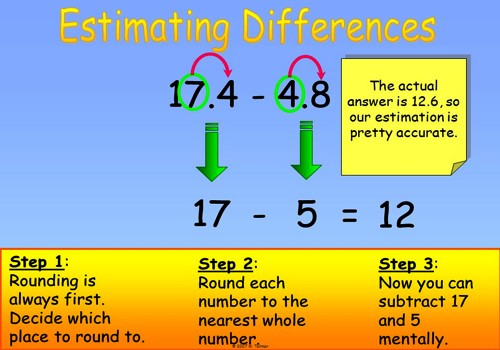Estimating a difference by rounding

In math and in real life, you will come across many problems where you need to find the difference. For instance, when you go shopping and you use a coupon for \$10 off your purchase of \$50 or more, in order to find out your total amount due, you need to find the difference. You need to subtract the \$10 from the amount that you are purchasing. So difference problems are really just subtraction problems.

You can estimate to find out “about how many” when subtracting as well as when adding.  This is also called rounding. You can estimate the differences of two-digit and three-digit numbers.

A quick way to estimate the difference between two numbers is to round each number and then subtract the rounded numbers. This probably won’t be the exact answer but it may be close enough for some purposes.

How to Estimate a difference by rounding.

• Round each term that will be subtracted
• Subtract the rounded numbersAn estimate can sometimes be improved. If the sum of 645 – 450 were estimated, we would round 645 to 600 and 450 to 500. The estimate would be 600 – 500 or 100. One number was rounded down and the other was rounded up. The number 645 was rounded down by 45 and 450 was rounded up by 50. Adding 45 + 50 gives 95, which rounds to 100. Therefore, a better estimate would be 200. The actual difference is 195.

How to Improve the Estimate.

• Round each term that will be subtracted
• Subtract the rounded numbers
• If one is rounded down and the other up see if the amount of rounding is more than 50. If it is add 100 to or subtract 100 from the estimate.
• If both numbers are rounded down or both are rounded up a closer estimate will not be produced by this method.

For example, rounding the number 16 to the tens place gives you 20. You round up because the number directly to the right of the digit that you are rounding – the 6, which is directly to the right of the 1 in the tens place – is 5 or greater. The 1 in the tens place changes to a 2. If our number is 13, then we round down by keeping our tens digit, the 1, and bringing the 3 down to get 0. We get 10.

Information Source: